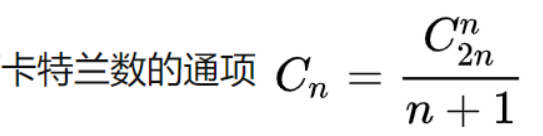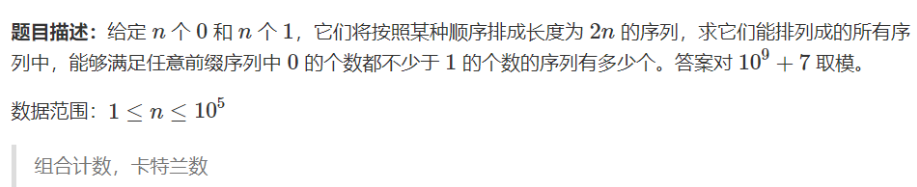# [数论](8)组合数的应用:卡特兰数

### 卡特兰数:C++ 将 01 序列置于坐标系中，起点定于原点。若 0 表示向右走，1 表示向上走，那么任何前缀中 0 的个数不少于 1 的个数就转化为：路径上的任意一点，横坐标大于等于纵坐标。 题目所求即为这样的合法路径数量。 下图中，表示从 (0,0) 走到 (n,n) 的路径，在绿线及以下表示合法，若触碰红线即不合法。


![image-20210519195925043](C:\Users\Axuan\AppData\Roaming\Typora\typora-user-images\image-20210519195925043.png)

C++

(需要注意:公式必须整理为最简,若采用C(2n,n)-C(2n,n-2)),当n>66时,即会爆long long

`C++

# include

using namespace std; typedef long long LL;

# define N 500010

const int p = 1e9+7; LL fact[N],infact[N]; LL qmi(LL a,LL k){//快速幂模板 LL res=1; while(k){ if(k&1) res=resa%p; k>>=1; a=aa%p; } return res; } void init(){//预处理阶乘 fact=infact=1; for(int i=1;i<N;i++){ fact[i]=ifact[i-1]%p; infact[i]=infact[i-1]qmi(i,p-2)%p; } return; } int main(){ init(); int n; scanf("%d",&n); int a=2n,b=n; //卡特兰数 LL ans=fact[a]infact[a-b]%pinfact[b]%pqmi(n+1,p-2); cout<<ans%p<<endl; return 0; }

## 本文链接：

https://nullcode.fun/146.html
1 + 4 =